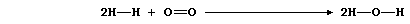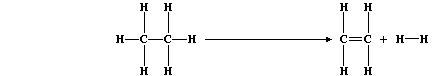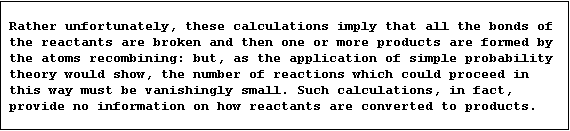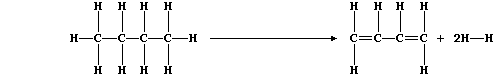```SELECTED PRINCIPLES: CHEMICAL ENERGETICS (1)
During a chemical reaction, bonds of the reactants are broken and then
those of the products are formed. Bond breaking is an endothermic or,
more generally, an endergonic process: by contrast, bond forming is an
exothermic or, more generally, an exergonic process. Each bond type has
its own bond energy, defined as 'the amount of energy needed to break
one mole of the bond or released when one mole of the bond is formed';
some typical values are shown in the Table immediately below.```
 ` Bond type` ` O-O` ` C-C` ` C-H` ` H-H` ` O-H` ` O=O` ` C=C` ` CļC ` ` Bond energy / kJ mol-Ļ` ` 146` ` 346` ` 413` ` 432` ` 463` ` 497` ` 610` ` 815`
```The heat energy change (DH) for a chemical reaction can be calculated
using bond energies - as these two exemplars illustrate.
The equation for the combustion of dihydrogen can be written as:```` Bonds broken ` ``` Energy absorbed / kJ mol-Ļ``` ` Bonds formed ` ``` Energy released / kJ mol-Ļ``` ` 2 H-H ` ` 864` ` 4 O-H ` ` 1852` ` 1 O=O ` ` 497` ` ` ` ` ` Total = 1361` ` Total = 1852`
```Energy absorbed - energy released = 1361 - 1852 = DH -491 kJ mol-¹.
Thus, in an exothermic reaction, 491 kJ of energy are released to the
surroundings when 2 moles of dihydrogen combust; i.e., 245.5 kJ mol-Ļ
of dihydrogen. [This combustion is most familiar as the explosive 'pop'
observed when testing for hydrogen gas with a lighted splint.]
The equation for the dehydrogenation of ethane can be written as:```` Bonds broken ` ``` Energy absorbed / kJ mol-Ļ``` ` Bonds formed ` ``` Energy released / kJ mol-Ļ``` ` 6 C-H ` ` 2478` ` 4 C-H ` ` 1652` ` 1 C-C ` ` 436` ` 1 C=C ` ` 610` ` ` ` ` ` 1 H-H` ` 432` ` Total = 2824` ` Total = 2694`
```Energy absorbed - energy released = 2824 - 2694 = DH +130 kJ mol-¹.
Thus, in an endothermic reaction, 130 kJ of energy are absorbed from
the surroundings when 1 mole of ethane dehydrogenates. [This reaction,
incidentally, is one of the principal methods by which dihydrogen is
obtained on an industrial scale.]``````1.  Dehydrogenation reactions are used in industry to obtain a range of
chemicals. Butadiene, for example, can be obtained by dehydrogenation
of butane; the equation for this reaction can be written as:````(a) Use the appropriate bond energies to complete this Table,`
 ` Bonds broken ` ``` Energy absorbed / kJ mol-Ļ``` ` Bonds formed ` ``` Energy released / kJ mol-Ļ``` ` ` ` ` ` ` ` ` ` ` ` ` ` ` ` ` ` ` ` ` ` ` ` ` ` ` ` ` ` ` ` ` ` Total =` ` Total =`
```                                                                    
in order to show that the heat energy change (DH) for this endothermic
reaction is +260 kJ mol-Ļ. ____________________________________________
_______________________________________________________________________

(b) Butadiene is a monomer used to synthesize various polymers; these,
in turn, are used to manufacture a variety of synthetic rubbers (known
as elastomers). Suggest the chemical name of one polymer derived from

2.  Hydrogenation reactions are, unsurprisingly, the chemical opposites
of dehydrogenation reactions. Ethane, for example, can be obtained by
hydrogenation of ethene; the equation for this reaction can be written
as:````Use the appropriate bond energies to complete this Table,`
 ` Bonds broken ` ``` Energy absorbed / kJ mol-Ļ``` ` Bonds formed ` ``` Energy released / kJ mol-Ļ``` ` ` ` ` ` ` ` ` ` ` ` ` ` ` ` ` ` ` ` ` ` ` ` ` ` Total = ` ` Total = `
```                                                                    
in order to show that the heat energy change (DH) for this exothermic
reaction is -130 kJ mol-Ļ. ____________________________________________
_______________________________________________________________________

3.  The hydrogenation of ethene to ethane is an exothermic reaction
with DH of -130 kJ mol-Ļ: whereas, the dehydrogenation of ethane to
ethene is an endothermic reaction with DH of +130 kJ mol-Ļ. This
'mirror-symmetry' is consistent with the Principle of the Conservation
of Energy, which states that 'energy is neither created nor destroyed,
but can be transduced from one form to another'. Noting that DH for the
dehydrogenation of butane to butadiene is +260 kJ mol-Ļ, what must
be DH for the hydrogenation of butadiene to butane? __________________

Dr. R. Peters                   Next                     Contents' List```